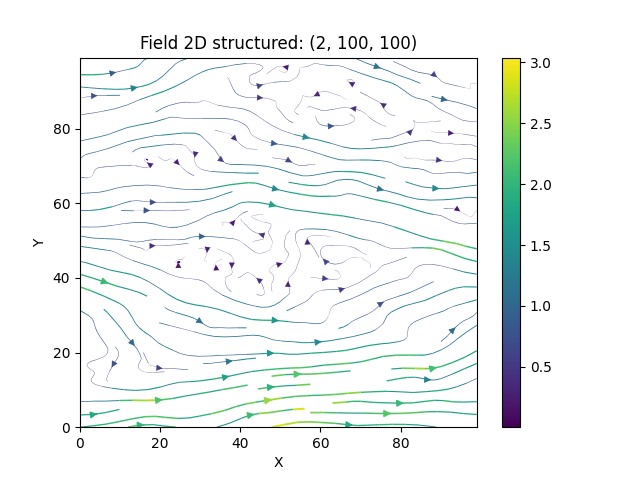Generating a Random 2D Vector Field

As a first example we are going to generate a 2d vector field with a Gaussian covariance model on a structured grid:

import numpy as np

import gstools as gs

# the grid
x = np.arange(100)
y = np.arange(100)

# a smooth Gaussian covariance model
model = gs.Gaussian(dim=2, var=1, len_scale=10)
srf = gs.SRF(model, generator="VectorField", seed=19841203)
srf((x, y), mesh_type="structured")
srf.plot()Let us have a look at the influence of the covariance model. Choosing the exponential model and keeping all other parameters the same

# a rougher exponential covariance model
model2 = gs.Exponential(dim=2, var=1, len_scale=10)
srf.model = model2
srf((x, y), mesh_type="structured", seed=19841203)
srf.plot()and we see, that the wiggles are much “rougher” than the smooth Gaussian ones.

Applications

One great advantage of the Kraichnan method is, that after some initializations, one can compute the velocity field at arbitrary points, online, with hardly any overhead. This means, that for a Lagrangian transport simulation for example, the velocity can be evaluated at each particle position very efficiently and without any interpolation. These field interpolations are a common problem for Lagrangian methods.

Total running time of the script: ( 0 minutes 2.592 seconds)

Gallery generated by Sphinx-Gallery